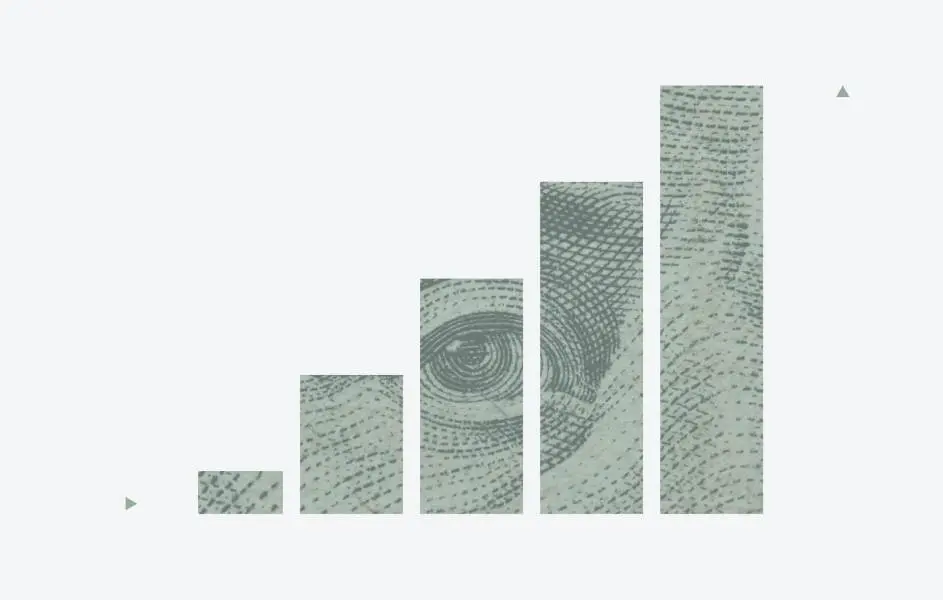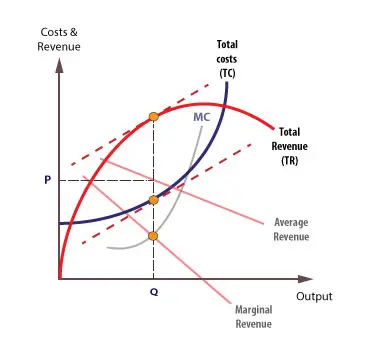# Profits

Profit has several meanings in economics. At its most basic level, profit is the reward gained by risk taking entrepreneurs when the revenue earned from selling a given amount of output exceeds the total costs of producing that output. This simple statement is often expressed as the profit identity, which states that:

##### Total profits = total revenue (TR) – total costs (TC)

However, the concept of profit needs clarification because there is no standard definition of what counts as a cost.

#### Normal profit

In markets which are perfectly competitive, the profit available to a single firm in the long run is called normal profit.  This exists when total revenue, TR, equals total cost, TC. Normal profit is defined as the minimum reward that is just sufficient to keep the entrepreneur supplying their enterprise. In other words, the reward is just covering opportunity cost – that is, just better than the next best alternative.

The accounting definition of profits is rather different because the calculation of profits is based on a straightforward numerical calculation of past monetary costs and revenues, and makes no reference to the concept of opportunity cost. Accounting profit occurs when revenues are greater than costs, and not equal, as in the case of normal profit.

To the economist, normal profit is a cost and is included in the total costs of production.

#### Super-normal (economic) profit

If a firm makes more than normal profit it is called super-normal profit. Supernormal profit is also called economic profit, and abnormal profit, and is earned when total revenue is greater than the total costs. Total costs include a reward to all the factors, including normal profit. This means that, when total revenue equals total cost, the entrepreneur is earning normal profit, which is the minimum reward that keeps the entrepreneur providing their skill, and taking risks.

The level of super-normal profits available to a firm is largely determined by the level of competition in a market – the more competition the less chance there is to earn super-normal profits.

Super-normal profit can be derived in three general cases:

1. By firms in perfectly competitive markets in the short run, before new entrants have eroded their profits down to a normal level.
2. By firms in less than competitive markets, like firms operating under monopolistic competition and competitive oligopolies, by innovating or reducing costs, and earning head start profits. These will eventually be eroded away, providing further incentive to innovate and become more cost efficient.
3. By firms in highly uncompetitive markets, like collusive oligopolies and monopolies, who can erect barriers to entry protect themselves from competition in the long run and earn persistent above normal profits.

#### Marginal profits

Marginal profit is the additional profit from selling one extra unit. A profit per unit will be achieved when marginal revenue (MR) is greater than marginal cost (MC). At profit maximisation, marginal profit is zero because MC = MR.

### Profit maximisation

Firms achieve maximum profits when marginal revenue (MR) is equal to marginal cost (MC), that is when the cost of producing one more unit of a good or service is exactly equal to the revenue derived from selling one extra unit.#### If marginal profit is greater than zero

If the firm stops short of producing Q, (at Q1 below) then MR is greater than MC, and marginal profit is still greater than zero. Hence, the firm should increase output.

#### If marginal profit is less than zero

If the firm produces greater than Q, (at Q2 below) MC is greater than MR and marginal profit is negative. Hence, the firm should reduce its output. Only when MR = MC, at Q, will total profits be maximised.### Showing profit maximisation using ‘totals’

Profit maximisation occurs at Q, given that the gap between total revenue (TR) and total costs (TC) is at its greatest. At this point, the gradient of the cost and revenue curves will be identical.Not all firms are profit maximisers. Profit maximisation is the most likely objective for a firm whose owners are involved in day-to-day decision making, such as with small and medium sized enterprises (SMEs).

## The number of firms and profits

The fewer the number of firms in a market, the less competitive it is likely to be.

### The effect of fewer firms:

Assuming that firms are selling substitute products, the effect of fewer firms is less competition, which will:

1. Reduce elasticity of demand.
2. Create steeper AR and MR curves.
3. Increase the level of supernormal profits for each firm.

## Profits and competition

Less competition in a given market is likely to lead to higher prices and the possibility of higher super-normal profits.

### Distributed and retained profits

When profits are generated, they can be retained by the firm, or distributed to its owners. A government may provide tax incentives for those firms that retain their profits, and use them for investment. Distributed profits are generally subject to corporation tax.